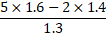# Quiz Discussion

$$\frac{{0.0203 \times 2.92}}{{0.0073 \times 14.5 \times 0.7}} = ?$$

Course Name: Quantitative Aptitude

• 1] 0.8
• 2] 1.45
• 3] 2.40
• 4] 3.25
##### Solution
No Solution Present Yet

#### Top 5 Similar Quiz - Based On AI&ML

Quiz Recommendation System API Link - https://fresherbell-quiz-api.herokuapp.com/fresherbell_quiz_api

# Quiz
1
Discuss

Which of the following is equal to 3.14 x 106 ?

• 1] 314
• 2] 3140
• 3] 3140000
• 4] None of these
##### Solution
2
Discuss

Which of the following are in descending order of their value ?

• 1]

$$\frac{1}{3},\frac{2}{5},\frac{3}{7},\frac{4}{5},\frac{5}{6},\frac{6}{7}$$

• 2]

$$\frac{1}{3},\frac{2}{5},\frac{3}{5},\frac{4}{7},\frac{5}{6},\frac{6}{7}$$

• 3]

$$\frac{1}{3},\frac{2}{5},\frac{3}{5},\frac{4}{6},\frac{5}{7},\frac{6}{7}$$

• 4]

$$\frac{6}{7},\frac{5}{6},\frac{4}{5},\frac{3}{7},\frac{2}{5},\frac{1}{3}$$

##### Solution
3
Discuss

The expression (11.98 × 11.98 + 11.98 × X + 0.02 × 0.02) will be a perfect square for X equal to:

• 1] 0.02
• 2] 0.2
• 3] 0.04
• 4] 0.4
##### Solution
4
Discuss

$$\frac{{\left( {0.1667} \right)\left( {0.8333} \right)\left( {0.3333} \right)}}{{\left( {0.2222} \right)\left( {0.6667} \right)\left( {0.1250} \right)}}$$     is approximately equal to:

• 1] 2
• 2] 2.40
• 3] 2.43
• 4] 2.50
##### Solution
5
Discuss= ?

• 1] 0.4
• 2] 1.2
• 3] 1.4
• 4] 4
##### Solution
6
Discuss

3889 + 12.952 - ? = 3854.002

• 1] 47.095
• 2] 47.752
• 3] 47.932
• 4] 47.95
##### Solution
7
Discuss

The rational number for recurring decimal 0.125125.... is:

• 1]

63/487

• 2]

119/993

• 3]

125/999

• 4]

None of these

##### Solution
8
Discuss

The rational number for the recurring decimal 0.125125..... is :

• 1]

63/487

• 2]

119/993

• 3]

125/999

• 4]

None of these

##### Solution
9
Discuss

Solve : $$7\frac{1}{2} - \left[ {2\frac{1}{4} ÷ \left\{ {1\frac{1}{4} - \frac{1}{2}\left( {1\frac{1}{2} - \frac{1}{3} - \frac{1}{6}} \right)} \right\}} \right]$$       = ?

• 1]

2/9

• 2]

$$4\frac{1}{2}$$

• 3]

$$9\frac{1}{2}$$

• 4]

$$1\frac{77}{228}$$

##### Solution
10
Discuss

$$\frac{{4.2 \times 4.2 - 1.9 \times 1.9}}{{2.3 \times 6.1}}$$    is equal to:

• 1] 0.5
• 2] 1
• 3] 20
• 4] 22
# Quiz